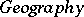# Coordinates

(diff) ← Older revision | Latest revision (diff) | Newer revision → (diff)

Numbers, quantities, used to specify (or determine) the position of some kind of element (point) in a collection (a set), e.g. on a plane or a surface, in a space, on a manifold. Coordinates are given different names in different branches of mathematics and physics; e.g. the coordinates of an element (vector) in a vector space are called its components, the coordinates in a product of sets are the projections on one of the factors; in relativity theory coordinate systems are frames of reference, etc. In many situations it is impossible to introduce sufficiently reasonable and convenient global coordinates on the entire set (e.g. the points of a sphere, unlike those of a plane, cannot be put in a continuous one-to-one correspondence with pairs of numbers), and one then introduces the concept of local coordinates. This, for example, is the situation in the theory of manifolds.

The set of coordinates is organized into a coordinate system (reference system), or a chart, with the coordinates corresponding in a one-to-one way with the elements of the set. This is the basis of the coordinate method, the roots of which are generally traced back to the work of P. Fermat (1636) and R. Descartes (1637). However, as long ago as the 3rd–2nd centuries B.C., Apollonius of Perga defined conic sections using what are now called coordinates (the term was coined by G. Leibniz, 1694), though Apollonius' coordinates did not have numerical values. By the 2nd century A.D., however, Ptolemy was already using numerical coordinates as latitude and longitude in his. In the 14th century, N. Oresme used coordinates on the plane to construct graphs, employing the terms longitude and latitude for what are now called abscissa and ordinate.

Attempts to avoid the introduction of coordinates "from without" , preserving as it were the "purity" of the theory, have not justified themselves (e.g. the synthetic constructions of projective coordinates cultivated by Ch. von Staudt (1847) proved to be replaceable by simple algebraic equivalents, a discovery that led to the concept of projective geometry over a division ring). Nevertheless, the idea still persists of a so to speak internal method of introducing coordinates (in contradistinction to the external method of imposing coordinates "from without" ), based on evaluating the position of the object to be provided with coordinates with respect to some a priori selected standard subsets, such as curves, surfaces and the like (which are then called coordinate curves, coordinate surfaces, etc.). This applies in particular to sets whose definitions involve numbers (e.g. metric and vector spaces), and therefore to a very broad range of mathematical objects of practical importance; this explains why such devices are so widespread.

A special position among coordinate systems for points (point coordinates) is occupied by linear coordinates, for which the coordinate curves are straight lines. Examples are a Cartesian orthogonal coordinate system, triangular coordinates (see Tetrahedral coordinates), barycentric coordinates, and projective coordinates. Coordinate systems for which not all coordinate curves are straight lines are known as curvilinear coordinates. Curvilinear coordinates are used both on the plane (e.g. polar coordinates; elliptic coordinates; parabolic coordinates; bipolar coordinates) and on surfaces (geodesic coordinates; isothermal coordinates and others). Many special types of curvilinear coordinate systems are introduced when one uses nets of curves satisfying various conditions. The most important class of such systems are orthogonal systems (cf. Orthogonal system), in which the coordinate curves intersect at right angles.

Various types of coordinates on the plane (or on a surface) admit a generalization to a (three-dimensional) space. For example, polar coordinates on the plane yield the concept of polar coordinates in space (spherical coordinates or cylinder coordinates); bipolar coordinates on the plane lead to the concepts of toroidal coordinates; bicylindrical coordinates and bipolar coordinates in space; elliptic coordinates on the plane yield the concept of ellipsoidal coordinates in space.

Considerations of convenience and clarity sometimes lead one to deviate from the familiar type of coordinates, in which the number of quantities constituting the coordinates of points in a set is equal to the dimension of that set. For similar reasons, the coordinate mapping may fail to be one-to-one at isolated points (this is the case, e.g., for polar coordinates).

In cases in which the manifoldunder consideration is not homeomorphic to a domain in a Euclidean space, it is sometimes convenient to use redundant coordinates, in which the number of coordinates exceeds the dimension of. Such coordinates are, usually, homogeneous coordinates.

One frequently speaks of the coordinates of straight lines, planes and other geometric objects, meaning thereby coordinates in some space whose "points" are straight lines, planes, etc. (see, e.g., Grassmann manifold). Since, according to the duality principle, points and straight lines in two-dimensional geometry are equivalent, it becomes possible to introduce coordinates which specify the positions of straight lines and planes. Example: tangential coordinates.

The coordinate method has become useful not only as a means toward the algorithmization of reasoning (reducing it to computation) but also for the discovery of new facts and relationships (for example, the consistency of Euclidean geometry is reduced via the use of coordinates to the consistency of arithmetic). And despite the face that many branches of mathematics, such as Riemannian geometry, can be presented in a "coordinate-free" form, the results themselves are most frequently achieved by the coordinate method, or — more precisely — via the judicious choice of coordinate systems for the specific problem at hand (for example, the significance of certain problems in mechanics is appreciated in terms of special coordinates, relative to which the variables are "separated" ).

How to Cite This Entry:
Coordinates. Encyclopedia of Mathematics. URL: http://encyclopediaofmath.org/index.php?title=Coordinates&oldid=31524
This article was adapted from an original article by M.I. VoitsekhovskiiA.B. Ivanov (originator), which appeared in Encyclopedia of Mathematics - ISBN 1402006098. See original article